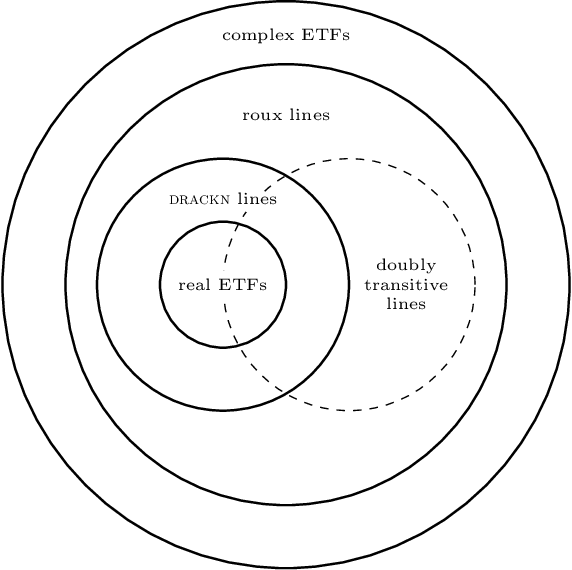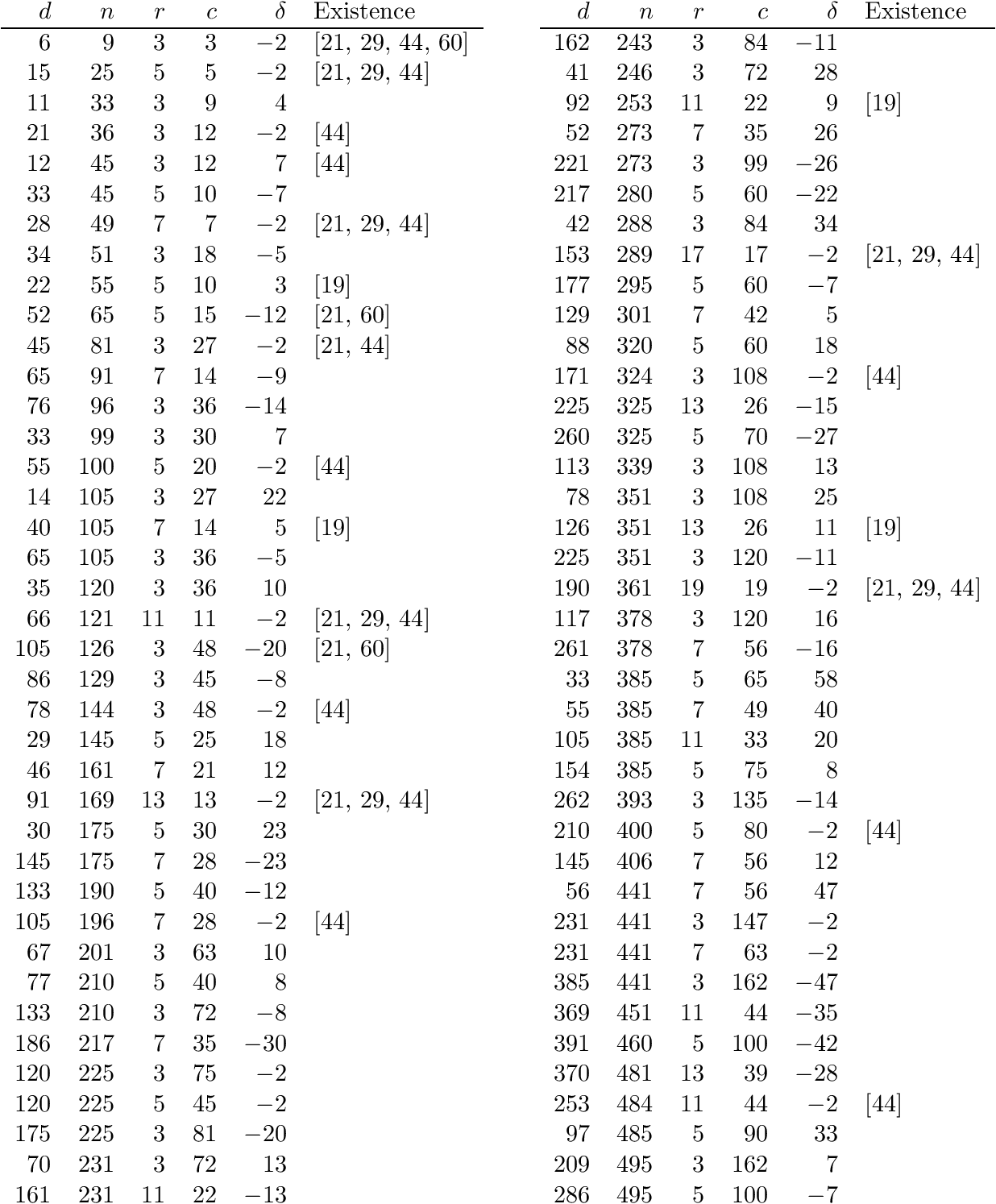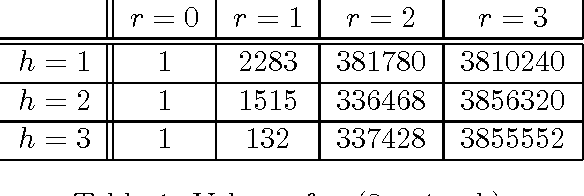Skip to search formSkip to main content
You are currently offline. Some features of the site may not work correctly.

# Adjacency algebra

In algebraic graph theory, the adjacency algebra of a graph G is the algebra of polynomials in the adjacency matrix A(G) of the graph. It is an… Expand
Wikipedia

## Papers overview

Semantic Scholar uses AI to extract papers important to this topic.
2019
2019
• J. Comb. Theory, Ser. A
• 2019
• Corpus ID: 119621834
Let $\Gamma$ denote a finite, undirected, connected graph, with vertex set $X$. Fix a vertex $x \in X$. Associated with $x$ is a… Expand
2018
2018
• ArXiv
• 2018
• Corpus ID: 49407494
We study lines through the origin of finite-dimensional complex vector spaces that enjoy a doubly transitive automorphism group… Expand
••2015
2015
• 2015
• Corpus ID: 55847102
Abstract The adjacency algebra of an association scheme is defined over an arbitrary field. In general, it is always semisimple… Expand
2014
2014
We compare the duality theories of rank-metric codes proposed by Delsarte and Gabidulin, proving that the former generalizes the… Expand
•2010
2010
• Electron. J. Comb.
• 2010
• Corpus ID: 18788172
It is well known that the complex adjacency algebra $A$ of an association scheme has a specific module, namely the standard… Expand
2004
2004
To each association scheme G and to each field R, there is associated naturally an associative algebra, the so-called adjacency… Expand
1999
1999
• Electron. J. Comb.
• 1999
• Corpus ID: 10763435
We define and study $m$-closed cellular algebras (coherent configurations) and $m$-isomorphisms of cellular algebras which can be… Expand
1987
1987
Abstract We begin with a discussion of orbit polynomial graphs and their relationship with distance-transitive, distance-regular… Expand
1986
1986
In order to study graphs, the notion of graph must first be defined. A graph is a set of points (called vertices, or nodes) and a… Expand
Highly Cited
1978
Highly Cited
1978
• P. Delsarte
• J. Comb. Theory, Ser. A
• 1978
• Corpus ID: 36235886
Abstract Let Ω be the set of bilinear forms on a pair of finite-dimensional vector spaces over GF(q). If two bilinear forms are… Expand